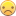## ODS PDF Won't Display Proc SGPLOT Chart

I have a simple daily dashboard I distribute via PDF and I was asked to add a chart to visualize one of the tables.  The Proc SGPLOt statement below works for me by itself, but when I wrap it in the ODS PDF statement, I get a blank page in the PDF.  When I run the SGPLOT by itself in the ODS PDF statement (i.e. getting rid of the proc print statements) I get some results, albeit they do look different on the PDF compared to being run outside of a PDF statement).  Is there anyway to get this to work?  Ideally, I would like to keep the first two tables on one page and then have one table and a chart on the second page.  I thought the conflict might be page size limitations, so I moved the SGPLOT to it's own page, with the same (blank page) results.  Any assistance is greatly appreciated.  Thanks!

ods listing;

goptions reset=all;

options date nobyline nonumber orientation=landscape;

ods pdf file='/filelocation/Dashboard.pdf' startpage = never style= BarrettsBlue;

proc print data=mydata1 noobs contents=""; run;

ods proclabel ' ';

ods escapechar='^';

proc print data=mydata2 noobs contents=""; run;

ods pdf startpage=now;

proc print data=mydata3 noobs contents=""; run;

ods pdf startpage=now;

PROC SGPLOT DATA = MyData3 description="";

SERIES X = Week Y = Group1 / lineattrs=(color=VIGB thickness=2) markers markerattrs=(symbol=asterisk color=VIGB);

SERIES X = Week Y = Group2 / lineattrs=(color=MAROON thickness=2) markers markerattrs=(symbol=circle color=MAROON);

SERIES X = Week Y = Group3 / lineattrs=(color=DELG thickness=2) markers markerattrs=(symbol=triangle color=DELG);

XAXIS TYPE = DISCRETE;

RUN;

ods pdf close;

5 REPLIES 5

## Re: ODS PDF Won't Display Proc SGPLOT Chart

do you have

ODS GRAPHICS ON; somewhere

or by default?

## Re: ODS PDF Won't Display Proc SGPLOT Chart

No, do I need that for the PDF?  Even after adding that, I still get the blank page.

## Re: ODS PDF Won't Display Proc SGPLOT Chart

Does the following work?

Works fine for meods listing;

goptions reset=all;

options date nobyline nonumber orientation=landscape;

ods pdf file='C:\_localdata\Dashboard.pdf' startpage = never style= BarrettsBlue;

proc print data=sashelp.class noobs contents=""; run;

ods proclabel ' ';

ods escapechar='^';

proc print data=sashelp.class noobs contents=""; run;

ods pdf startpage=now;

proc print data=sashelp.class noobs contents=""; run;

ods pdf startpage=now;

PROC SGPLOT DATA = sashelp.stocks description="";

SERIES X = date Y = high / lineattrs=(color=VIGB thickness=2) markers markerattrs=(symbol=asterisk color=VIGB);

SERIES X = date Y = low / lineattrs=(color=MAROON thickness=2) markers markerattrs=(symbol=circle color=MAROON);

SERIES X = date Y = open / lineattrs=(color=DELG thickness=2) markers markerattrs=(symbol=triangle color=DELG);

XAXIS TYPE = DISCRETE;

RUN;

ods pdf close;

## Re: ODS PDF Won't Display Proc SGPLOT Chart

Nope, same thing.  I get the first two tables on one page, a 3rd table on the 2nd page and a blank page where the graph should be.

## Re: ODS PDF Won't Display Proc SGPLOT Chart

Note: Goptions doesn't have a lot of affect on SG procedures. ODS Graphics options would be what may need resetting.

Are you getting any warning or messages in the log?
Try setting the height and width using ODS Graphics /height=5in width=5in; or similar size in case the default is trying to use more space than the PDF display area allows.

Discussion stats
• 5 replies
• 3417 views
• 0 likes
• 3 in conversation